# CIE 1931 color space

CIE 1931 color space

In the study of color perception, one of the first mathematically defined color spaces is the CIE 1931 XYZ color space, created by the International Commission on Illumination (CIE) in 1931.

The CIE XYZ color space was derived from a series of experiments done in the late 1920s by W. David Wright and John Guild. Their experimental results were combined into the specification of the CIE RGB color space, from which the CIE XYZ color space was derived.

## Tristimulus values

The human eye has photoreceptors (called cone cells) for medium- and high-brightness color vision, with sensitivity peaks in short (S, 420–440 nm), middle (M, 530–540 nm), and long (L, 560–580 nm) wavelengths (there are also the low-brightness, monochromatic "night-vision" receptors, called rod cells, which have peak sensitivity around 490–495 nm). Thus, in principle, three parameters describe a color sensation. The tristimulus values of a color are the amounts of three primary colors in a three-component additive color model needed to match that test color. The tristimulus values are most often given in the CIE 1931 color space, in which they are denoted X, Y, and Z.

Any specific method for associating tristimulus values with each color is called a color space. CIE XYZ, one of many such spaces, is a commonly used standard, and serves as the basis from which many other color spaces are defined.

## The CIE standard observer

In the CIE XYZ color space, the tristimulus values are not the S, M, and L responses of the human eye, but rather a set of tristimulus values called X, Y, and Z, which are roughly red, green and blue, respectively (note that the X,Y,Z values are not physically observed red, green, blue colors. Rather, they may be thought of as 'derived' parameters from the red, green, blue colors). Two light sources, made up of different mixtures of various wavelengths, may appear to be the same color; this effect is called metamerism. Two light sources have the same apparent color to an observer when they have the same tristimulus values, no matter what spectral distributions of light were used to produce them.

Due to the distribution of cones in the eye, the tristimulus values depend on the observer's field of view. To eliminate this variable, the CIE defined the standard (colorimetric) observer. Originally this was taken to be the chromatic response of the average human viewing through a 2° angle, due to the belief that the color-sensitive cones resided within a 2° arc of the fovea. Thus the CIE 1931 Standard Observer is also known as the CIE 1931 2° Standard Observer. A more modern but less-used alternative is the CIE 1964 10° Standard Observer, which is derived from the work of Stiles and Burch, and Speranskaya.

For the 10° experiments, the observers were instructed to ignore the central 2° spot. The 1964 Supplementary Standard Observer is recommended for more than about a 4° field of view. Both standard observers are discretized at 5 nm wavelength intervals and distributed by the CIE.

The standard observer is characterized by three color matching functions.

The derivation of the CIE standard observer from color matching experiments is given below, after the description of the CIE RGB space.

### Color matching functions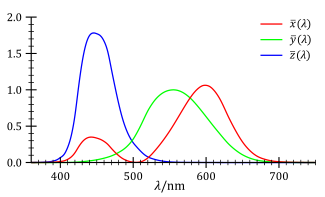The CIE standard observer color matching functions

The color matching functions are the numerical description of the chromatic response of the observer (described above).

The CIE has defined a set of three color-matching functions, called$\overline{x}(\lambda)$,$\overline{y}(\lambda)$, and$\overline{z}(\lambda)$, which can be thought of as the spectral sensitivity curves of three linear light detectors that yield the CIE XYZ tristimulus values X, Y, and Z. The tabulated numerical values of these functions are known collectively as the CIE standard observer.

The tristimulus values for a color with a spectral power distribution$I(\lambda)\,$ are given in terms of the standard observer by:$X= \int_0^\infty I(\lambda)\,\overline{x}(\lambda)\,d\lambda$$Y= \int_0^\infty I(\lambda)\,\overline{y}(\lambda)\,d\lambda$$Z= \int_0^\infty I(\lambda)\,\overline{z}(\lambda)\,d\lambda$

where$\lambda\,$ is the wavelength of the equivalent monochromatic light (measured in nanometers).

Other observers, such as for the CIE RGB space or other RGB color spaces, are defined by other sets of three color-matching functions, and lead to tristimulus values in those other spaces.

The values of X, Y, and Z are bounded if the intensity spectrum I(λ) is bounded.

## The CIE xy chromaticity diagram and the CIE xyY color space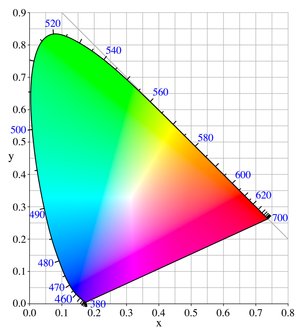The CIE 1931 color space chromaticity diagram. The outer curved boundary is the spectral (or monochromatic) locus, with wavelengths shown in nanometers. Note that the image itself describes colors using sRGB, and colors outside the sRGB gamut cannot be displayed properly. Depending on the color space and calibration of your display device, the sRGB colors may not be displayed properly either. This diagram displays the maximallly saturated bright colors that can be produced by a computer monitor or television set.The CIE 1931 color space chromaticity diagram rendered in terms of the colors of lower saturation and value than those displayed in the diagram above that can be produced by pigments, such as those used in printing. The color names are from the Munsell color system.

Since the human eye has three types of color sensors that respond to different ranges of wavelengths, a full plot of all visible colors is a three-dimensional figure. However, the concept of color can be divided into two parts: brightness and chromaticity. For example, the color white is a bright color, while the color grey is considered to be a less bright version of that same white. In other words, the chromaticity of white and grey are the same while their brightness differs.

The CIE XYZ color space was deliberately designed so that the Y parameter was a measure of the brightness or luminance of a color. The chromaticity of a color was then specified by the two derived parameters x and y, two of the three normalized values which are functions of all three tristimulus values X, Y, and Z:$x = \frac{X}{X+Y+Z}$$y = \frac{Y}{X+Y+Z}$$z = \frac{Z}{X+Y+Z} = 1 - x - y$

The derived color space specified by x, y, and Y is known as the CIE xyY color space and is widely used to specify colors in practice.

The X and Z tristimulus values can be calculated back from the chromaticity values x and y and the Y tristimulus value:$X=\frac{Y}{y}x$$Z=\frac{Y}{y}(1-x-y)$

The figure on the right shows the related chromaticity diagram. The outer curved boundary is the spectral locus, with wavelengths shown in nanometers. Note that the chromaticity diagram is a tool to specify how the human eye will experience light with a given spectrum. It cannot specify colors of objects (or printing inks), since the chromaticity observed while looking at an object depends on the light source as well.

Mathematically, x and y are projective coordinates and the colors of the chromaticity diagram occupy a region of the real projective plane.

The chromaticity diagram illustrates a number of interesting properties of the CIE XYZ color space:

• The diagram represents all of the chromaticities visible to the average person. These are shown in color and this region is called the gamut of human vision. The gamut of all visible chromaticities on the CIE plot is the tongue-shaped or horseshoe-shaped figure shown in color. The curved edge of the gamut is called the spectral locus and corresponds to monochromatic light (each point representing a pure hue of a single wavelength), with wavelengths listed in nanometers. The straight edge on the lower part of the gamut is called the line of purples. These colors, although they are on the border of the gamut, have no counterpart in monochromatic light. Less saturated colors appear in the interior of the figure with white at the center.
• It is seen that all visible chromaticities correspond to non-negative values of x, y, and z (and therefore to non-negative values of X, Y, and Z).
• If one chooses any two points of color on the chromaticity diagram, then all the colors that lie in a straight line between the two points can be formed by mixing these two colors. It follows that the gamut of colors must be convex in shape. All colors that can be formed by mixing three sources are found inside the triangle formed by the source points on the chromaticity diagram (and so on for multiple sources).
• An equal mixture of two equally bright colors will not generally lie on the midpoint of that line segment. In more general terms, a distance on the xy chromaticity diagram does not correspond to the degree of difference between two colors. In the early 1940s, David MacAdam studied the nature of visual sensitivity to color differences, and summarized his results in the concept of a MacAdam ellipse. Based on the work of MacAdam, the CIE 1960, CIE 1964, and CIE 1976 color spaces were developed, with the goal of achieving perceptual uniformity (have an equal distance in the color space correspond to equal differences in color). Although they were a distinct improvement over the CIE 1931 system, they were not completely free of distortion.
• It can be seen that, given three real sources, these sources cannot cover the gamut of human vision. Geometrically stated, there are no three points within the gamut that form a triangle that includes the entire gamut; or more simply, the gamut of human vision is not a triangle.
• Light with a flat power spectrum in terms of wavelength (equal power in every 1 nm interval) corresponds to the point (x,y) = (1/3,1/3).

## Definition of the CIE XYZ color space

### Experimental results: the CIE RGB color space

The CIE RGB color space is one of many RGB color spaces, distinguished by a particular set of monochromatic (single-wavelength) primary colors.

In the 1920s, W. David Wright and John Guild independently conducted a series of experiments on human sight which laid the foundation for the specification of the CIE XYZ color space.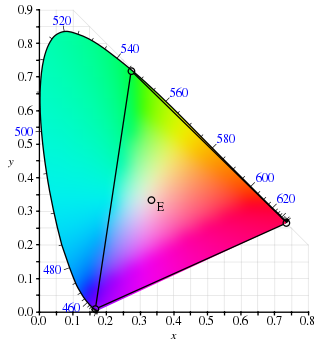Gamut of the CIE RGB primaries and location of primaries on the CIE 1931 xy chromaticity diagram.

The experiments were conducted by using a circular split screen 2 degrees in size, which is the angular size of the human fovea. On one side of the field a test color was projected and on the other side, an observer-adjustable color was projected. The adjustable color was a mixture of three primary colors, each with fixed chromaticity, but with adjustable brightness.

The observer would alter the brightness of each of the three primary beams until a match to the test color was observed. Not all test colors could be matched using this technique. When this was the case, a variable amount of one of the primaries could be added to the test color, and a match with the remaining two primaries was carried out with the variable color spot. For these cases, the amount of the primary added to the test color was considered to be a negative value. In this way, the entire range of human color perception could be covered. When the test colors were monochromatic, a plot could be made of the amount of each primary used as a function of the wavelength of the test color. These three functions are called the color matching functions for that particular experiment.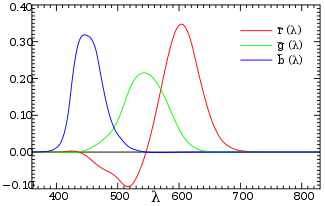The CIE 1931 RGB Color matching functions. The color matching functions are the amounts of primaries needed to match the monochromatic test primary at the wavelength shown on the horizontal scale.

Although Wright and Guild's experiments were carried out using various primaries at various intensities, and a number of different observers, all of their results were summarized by the standardized CIE RGB color matching functions$\overline{r}(\lambda)$,$\overline{g}(\lambda)$, and$\overline{b}(\lambda)$, obtained using three monochromatic primaries at standardized wavelengths of 700 nm (red), 546.1 nm (green) and 435.8 nm (blue). The color matching functions are the amounts of primaries needed to match the monochromatic test primary. These functions are shown in the plot on the right (CIE 1931). Note that$\overline{r}(\lambda)$ and$\overline{g}(\lambda)$ are zero at 435.8,$\overline{r}(\lambda)$ and$\overline{b}(\lambda)$ are zero at 546.1 and$\overline{g}(\lambda)$ and$\overline{b}(\lambda)$ are zero at 700 nm, since in these cases the test color is one of the primaries. The primaries with wavelengths 546.1 nm and 435.8 nm were chosen because they are easily reproducible monochromatic lines of a mercury vapor discharge. The 700 nm wavelength, which in 1931 was difficult to reproduce as a monochromatic beam, was chosen because the eye's perception of color is rather unchanging at this wavelength, and therefore small errors in wavelength of this primary would have little effect on the results.

The color matching functions and primaries were settled upon by a CIE special commission after considerable deliberation. The cut-offs at the short- and long-wavelength side of the diagram are chosen somewhat arbitrarily; the human eye can actually see light with wavelengths up to about 810 nm, but with a sensitivity that is many thousand times lower than for green light. These color matching functions define what is known as the "1931 CIE standard observer". Note that rather than specify the brightness of each primary, the curves are normalized to have constant area beneath them. This area is fixed to a particular value by specifying that$\int_0^\infty \overline{r}(\lambda)\,d\lambda= \int_0^\infty \overline{g}(\lambda)\,d\lambda= \int_0^\infty \overline{b}(\lambda)\,d\lambda$

The resulting normalized color matching functions are then scaled in the r:g:b ratio of 1:4.5907:0.0601 for source luminance and 72.0962:1.3791:1 for source radiant power to reproduce the true color matching functions. By proposing that the primaries be standardized, the CIE established an international system of objective color notation.

Given these scaled color matching functions, the RGB tristimulus values for a color with a spectral power distribution I(λ) would then be given by:$R= \int_0^\infty I(\lambda)\,\overline{r}(\lambda)\,d\lambda$$G= \int_0^\infty I(\lambda)\,\overline{g}(\lambda)\,d\lambda$$B= \int_0^\infty I(\lambda)\,\overline{b}(\lambda)\,d\lambda$

These are all inner products and can be thought of as a projection of an infinite-dimensional spectrum to a three-dimensional color. (See also: Hilbert space)

### Grassmann's law

One might ask: "Why is it possible that Wright and Guild's results can be summarized using different primaries and different intensities from those actually used?" One might also ask: "What about the case when the test colors being matched are not monochromatic?" The answer to both of these questions lies in the (near) linearity of human color perception. This linearity is expressed in Grassmann's law.

The CIE RGB space can be used to define chromaticity in the usual way: The chromaticity coordinates are r and g where:$r= \frac{R}{R+G+B},$$g= \frac{G}{R+G+B}.$

### Construction of the CIE XYZ color space from the Wright–Guild data

Having developed an RGB model of human vision using the CIE RGB matching functions, the members of the special commission wished to develop another color space that would relate to the CIE RGB color space. It was assumed that Grassmann's law held, and the new space would be related to the CIE RGB space by a linear transformation. The new space would be defined in terms of three new color matching functions$\overline{x}(\lambda)$,$\overline{y}(\lambda)$, and$\overline{z}(\lambda)$ as described above. The new color space would be chosen to have the following desirable properties: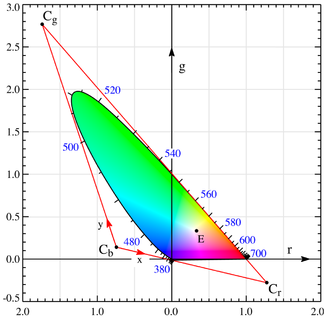Diagram in CIE rg chromaticity space showing the construction of the triangle specifying the CIE XYZ color space. The triangle Cb-Cg-Cr is just the xy=(0,0),(0,1),(1,0) triangle in CIE xy chromaticity space. The line connecting Cb and Cr is the alychne. Notice that the spectral locus passes through rg=(0,0) at 435.8 nm, through rg=(0,1) at 546.1 nm and through rg=(1,0) at 700 nm. Also, the equal energy point (E) is at rg=xy=(1/3,1/3).
1. The new color matching functions were to be everywhere greater than or equal to zero. In 1931, computations were done by hand or slide rule, and the specification of positive values was a useful computational simplification.
2. The$\overline{y}(\lambda)$ color matching function would be exactly equal to the photopic luminous efficiency function V(λ) for the "CIE standard photopic observer". The luminance function describes the variation of perceived brightness with wavelength. The fact that the luminance function could be constructed by a linear combination of the RGB color matching functions was not guaranteed by any means but might be expected to be nearly true due to the near-linear nature of human sight. Again, the main reason for this requirement was computational simplification.
3. For the constant energy white point, it was required that x = y = z = 1/3.
4. By virtue of the definition of chromaticity and the requirement of positive values of x and y, it can be seen that the gamut of all colors will lie inside the triangle [1,0], [0,0], [0,1]. It was required that the gamut fill this space practically completely.
5. It was found that the$\overline{z}(\lambda)$ color matching function could be set to zero above 650 nm while remaining within the bounds of experimental error. For computational simplicity, it was specified that this would be so.

In geometrical terms, choosing the new color space amounts to choosing a new triangle in rg chromaticity space. In the figure above-right, the rg chromaticity coordinates are shown on the two axes in black, along with the gamut of the 1931 standard observer. Shown in red are the CIE xy chromaticity axes which were determined by the above requirements. The requirement that the XYZ coordinates be non-negative means that the triangle formed by Cr, Cg, Cb must encompass the entire gamut of the standard observer. The line connecting Cr and Cb is fixed by the requirement that the$\overline{y}(\lambda)$ function be equal to the luminance function. This line is the line of zero luminance, and is called the alychne. The requirement that the$\overline{z}(\lambda)$ function be zero above 650 nm means that the line connecting Cg and Cr must be tangent to the gamut in the region of Kr. This defines the location of point Cr. The requirement that the equal energy point be defined by x = y = 1/3 puts a restriction on the line joining Cb and Cg, and finally, the requirement that the gamut fill the space puts a second restriction on this line to be very close to the gamut in the green region, which specifies the location of Cg and Cb. The above described transformation is a linear transformation from the CIE RGB space to XYZ space. The standardized transformation settled upon by the CIE special commission was as follows:

The numbers below all have the correct number of significant digits per CIE standards.$\begin{bmatrix}X\\Y\\Z\end{bmatrix}=\frac{1}{b_{21}} \begin{bmatrix} b_{11}&b_{12}&b_{13}\\ b_{21}&b_{22}&b_{23}\\ b_{31}&b_{32}&b_{33} \end{bmatrix} \begin{bmatrix}R\\G\\B\end{bmatrix}=\frac{1}{0.17697} \begin{bmatrix} 0.49&0.31&0.20\\ 0.17697&0.81240&0.01063\\ 0.00&0.01&0.99 \end{bmatrix} \begin{bmatrix}R\\G\\B\end{bmatrix}$

The integrals of the XYZ color matching functions must all be equal by requirement 3 above, and this is set by the integral of the photopic luminous efficiency function by requirement 2 above. The tabulated sensitivity curves have a certain amount of arbitrariness in them. The shapes of the individual X, Y and Z sensitivity curves can be measured with a reasonable accuracy. However, the overall luminosity curve (which in fact is a weighted sum of these three curves) is subjective, since it involves asking a test person whether two light sources have the same brightness, even if they are in completely different colors. Along the same lines, the relative magnitudes of the X, Y, and Z curves are arbitrary. One could as well define a valid color space with an X sensitivity curve that has twice the amplitude. This new color space would have a different shape. The sensitivity curves in the CIE 1931 and 1964 XYZ color spaces are scaled to have equal areas under the curves.

Wikimedia Foundation. 2010.

Нужна помощь с курсовой?

### Look at other dictionaries:

• CIE 1960 color space — The CIE 1960 color space ( CIE 1960 UCS , variously expanded Uniform Color Space , Uniform Color Scale , Uniform Chromaticity Scale , Uniform Chromaticity Space ) is another name for the (u,v) chromaticity space devised by David MacAdam. [cite… …   Wikipedia

• Color space — A comparison of the chromaticities enclosed by some color spaces. A color model is an abstract mathematical model describing the way colors can be represented as tuples of numbers, typically as three or four values or color components (e.g. RGB… …   Wikipedia

• ProPhoto RGB color space — The ProPhoto RGB color space, also known as ROMM RGB, is an output referred RGB color space, developed by Kodak, that offers an especially large gamut designed for use with photographic output in mind. The ProPhoto RGB color space encompasses… …   Wikipedia

• Lab color space — A Lab color space is a color opponent space with dimension L for lightness and a and b for the color opponent dimensions, based on nonlinearly compressed CIE XYZ color space coordinates.The coordinates of the Hunter 1948 L , a , b color space are …   Wikipedia

• RGB color space — An RGB color space is any additive color space based on the RGB color model. A particular RGB color space is defined by the three chromaticities of the red, green, and blue additive primaries, and can produce any chromaticity that is the triangle …   Wikipedia

• CIELUV color space — In colorimetry, the CIE 1976 ( L* , u* , v* ) color space, also known as the CIELUV color space, is a color space adopted by the International Commission on Illumination (CIE) in 1976, as a simple to compute transformation of the 1931 CIE XYZ… …   Wikipedia

• LMS color space — LMS is a color space represented by the response of the three types of cones of the human eye, named after their responsivity (sensitivity) at long, medium and short wavelengths.It is common to use the LMS color space when performing chromatic… …   Wikipedia

• Adams chromatic valence color space — Adams chromatic valence color spaces are a class of color spaces suggested by Elliot Quincy Adams.cite conference|title=Chromatic Valence as a Correlate of Munsell Chroma|authorlink=Elliot Quincy Adams|first=Elliot… …   Wikipedia

• CIE — is an acronym which can stand for:* Cambridge International Examinations, an international examination board * Commercial Information Exchange, an amalgamation of commercial real estate data * the International Commission on Illumination ** the… …   Wikipedia

• Color model — A color model is an abstract mathematical model describing the way colors can be represented as tuples of numbers, typically as three or four values or color components. When this model is associated with a precise description of how the… …   Wikipedia